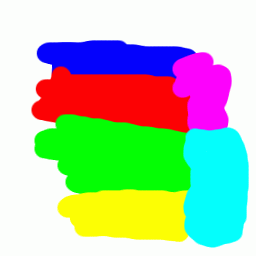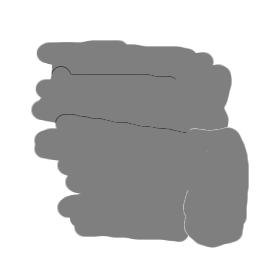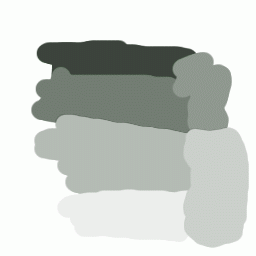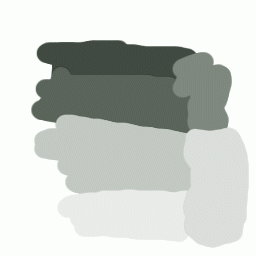# But, what is the REAL Y value of my monitor?

The Y value, a weighted sum of red,green,and blue, is a way to produce faithful representation of luminance, and can be used to convert an image to greyscale, amongst other things. Typical software uses an Y formula to convert a colour image to greyscale. This is NOT the same as `desaturation', which uses the HLS/HSV model to produce a greyscale image.

After a lot of experimenting with gammas and other stuff to improve my colours, I found out that the problems I've been having with saturating my pictures came from the fact that I was using the wrong Y formula! I got to the point of using a mathematically correct formula, and it was only then that I found out something was wrong here.

So, the traditional Y formula is:

```    Y-standard = R*0.299 + G*0.587 + B*0.114
```
You will find this equation in many docs and in various software. It is claimed that this correctly describes the luminance of typical computer monitors. Green is twice as bright as red, which is thrice as bright as blue.

I found that this is not so. It appears that monitors consistently produce darker reds than this formula suggests. The following formula, determined experimentally, looks significantly more correct (at least perceptually):

```    Y-improved = R*0.211 + G*0.658 + B*0.131
```
The following sample shows the difference. Move the mouse over the picture to see the original.Original`Desaturation' using L or V value.Standard YExperimentally improved Y